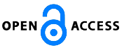Isaac Scientific Publishing### A New Iterational Method for Ordinary Equations Using Sumudu Transform

Download PDF (458.8 KB) PP. 89 - 94 Pub. Date: October 25, 2016

### Author(s)

• Yanqin Liu and Wen Chen
1Department of Engineering Mechanics, Hohai University, Jiangsu, 210098, China
2School of Mathematical Sciences, Dezhou University, Dezhou 253023, China

### Abstract

A novel modification of the variational iteration method (VIM) is proposed by means of the Sumudu transform. This approach is a universal way to identify the multiplier, which is simple and effective, and successfully extended to ordinary differential equations, including the nonlinear and variable coefficient ones. The Lagrange multiplier can be easily identified by the Sumudu transform and the nonlinear term will be handled by the Adomian series. Examples are given to elucidate the process and the reliability of the method.

### Keywords

Approximate solution, variational iteration method, Lagrange multiplier, Sumudu transform, nonlinear equation.

### References

 J.H. He, "Variational iteration method-a kind of nonlinear analytical technique: some examples", International Journal of Non-Linear Mechanics, Vol. 34 No. 4, pp. 699-708,1999.

 J.H. He, X.H. Wu, "Variational iteration method: new development and applications", Computers and Mathematics with Applications, Vol. 54 No.7-8, pp.881-894, 2007.

 J.H. He, "Asympototic methods for solitary solutions and compactons",Abstract and Applied Analysis, Vol. 2012, Article ID 916793, 2012.

 A.M. Wazwaz, "A comparison between the variational iteration method and Adomian decomposition method", Journal of Computational and Applied Mathematics, Vol. 207 No. 1, pp. 129-136, 2007.

 A.M. Wazwaz, "The variational iteration method for analytic treatment for linear and nonlinear ODEs", Applied Mathematics and Coputation, Vol. 212 No. 1, pp. 120-134, 2009.

 M.A. Noor, S.T. Mohyud-Din, "Variational iteration method for solving higher-order nonlinear boundary value problems using He’s polynomials", , Vol. 9 No. 2, pp. 141-156, 2008.

 M. A. Noor, "Some iterative methods for solving nonlinear equations using homotopy perturbation method", International Journal of Computer Mathematics", Vol. 87 No. 1, pp. 141-149, 2010.

 F. Geng, "A modified variational iteration method for solving Riccati differential equations", Computers and Mathematics with Applications,Vol. 60 No. 7, pp. 1868-1872, 2010.

 H. Jafari, C.M. Khalique, "Homotopy perturbation and variational iteration methods for solving fuzzy differential equations", Communications in Fractional Calculus, Vol. 3 No. 1, pp. 38-48, 2012.

 Y.Q. Liu, "Variational homotopy perturbation method for solving fractional initial boundary value problems", Abstract and Applied Analysis, Vol. 2012, Article ID 727031, 10 pages, 2012.

 Y.Q. Liu, F.S. Xu, X.L. Yin, "Variational approximate solutions of fractional nonlinear nonhomogeneous equations with laplace transform", Abstract and Applied Analysis,Vol. 2013, Article ID 819268, 9 pages, 2013.

 G.C. Wu, "Challenge in the variational iteration method - A new approach to identification of the lagrange multipliers", Journal of King Saud University-Science, Vol. 25 No. 2, pp. 175-178, 2013.

 G.C. Wu, "Laplace transform overcoming principle drawbacks in application of the variational iteration method to fractional heat equations", Thermal Science, Vol. 16 No. 4, pp. 1257-1261, 2012.

 G.C. Wu, D. Baleanu, "Variational iteration method for fractional calculus – a universal approach by laplace transform", Advances in Difference Equations, 2013: 18 pp. 1-9, 2013.

 G.C. Wu, D. Baleanu, "Variational iteration method for the Burgers’ flow with fractional derivatives- New Lagrange multipliers", Applied Mathematical Modelling, Vol. 37 No. 9, pp. 6183-6190, 2013.

 Abdon Atangana, Necdet Bildik, and S.C. Oukouomi Noutchie, "New iteration methods for time-fractional modified nonlinear kawahara equation", Abstract and Applied Analysis, Vol. 2014, Article ID 740248, 9 pages, 2014.

 Hasan Bulut, Haci Mehmet Baskonus, and Tethi Bin Muhammad Belgacem, "The analytical solution of some fractional ordinary differential equations by the sumudu transform method", Abstract and Applied Analysis, Vol. 2013, Article ID 203875, 6 pages, 2013.

 A.A. Elbeleze, A. kili?man, B.M. Taib, " Homotopy perturbation method for fractional Block-Scholes european option pricing equations using Sumudu transform", Mathematical Problems in Engineering, Vol. 2013, Article ID 524852, 7 pages, 2013.

 G.K. Watugala, "Sumudu transform–a new integral transform to solve differential equations and control engineering problems", Mathematical Engineering in Industry, Vol. 24 No. 1, pp. 35-43, 1993.

 G. Adomian, "A review of the decomposition method in applied mathematics", Journal of Mathematical Analysis and Applications, Vol. 135 No. 2, pp. 501-544, 1988.